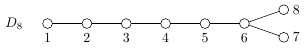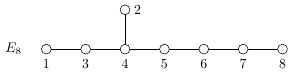Queries for Finite Cartan types: search statistic / browse statistics / browse maps from / browse maps to

# 1. Definition & Example

• An irreducible finite Cartan type is one of the following:

• $A_n$ for $n \geq 1$;

• $B_n$ for $n \geq 2$;

• $C_n$ for $n \geq 3$;

• $D_n$ for $n \geq 4$;

• $E_n$ for $n = 6,7,8$;

• $F_4$;

• $G_2$;

• The rank of an irreducible finite Cartan type is by its index. It denotes the dimension of the corresponding irreducible representation of its Weyl group.

 the 5 Finite Cartan types of size 8 ['A',8]['B',8]['C',8]['D',8]['E',8]## 2.1. Finite root systems

Let $V$ be an Euclidean vector space of finite dimension, endowed with an inner product $(\cdot, \cdot)$, and let $\alpha\in V$ with $\alpha \neq 0$. The reflection $s_\alpha:V\rightarrow V$ orthogonal to $\alpha$ is defined as $$s_\alpha(\lambda) = \lambda - 2\frac{(\lambda,\alpha)}{(\alpha,\alpha)}\alpha \text{ for }\lambda \in V.$$ A (crystallographic) root system in $V$ is a finite set $\Phi\subset V\setminus \{0\}$ that spans $V$ and satisfies

1. $\Phi \cap \mathbb{R}\alpha = \{\alpha, -\alpha\}$,

2. $s_\alpha \Phi = \Phi$ for all $\alpha \in \Phi$ and

3. $\langle\beta,\alpha\rangle := 2\frac{(\beta,\alpha)}{(\alpha,\alpha)} \in \mathbb{Z}$ for all $\alpha,\beta\in \Phi$.

The elements of $\Phi$ are called roots. The root system $\Phi$ is called reducible, if there is a disjoint union $\Phi = \Phi_1 \sqcup \Phi_2$ in nonempty sets such that $(\alpha,\beta) = 0$ for all $\alpha \in \Phi_1$ and $\beta \in \Phi_2$. Otherwise it is called irreducible.

Associated to a root system is its Weyl group $$W_\Phi = < s_\alpha \mid \alpha \in \Phi >\subset \operatorname{GL}(V)$$ that is generated by the reflections perpendicular to the roots of $\Phi$. The Weyl group is completely determined by the root system.

Let $\Phi$ be a root system. A subset $\Delta \subset \Phi$ is called a simple system if it is a basis of $V$ and every root in $\Phi$ is a linear combination of elements of $\Delta$ with integral coefficients all of the same sign (or zero).

Associated to a simple system $\Delta$ is the positive system $\Phi^+$ containing all roots that are linear combinations of $\Delta$ with only nonnegative coefficients.

Elements of $\Delta$ are called simple roots and elements of $\Phi^+$ are called positive roots. For every root system, simple systems exist, see [Hum72].

Let $\Delta = \{\alpha_1,\dots,\alpha_n\}$ be a simple system of $\Phi$. The Dynkin diagram of $\Phi$ is defined as a graph on $n$ vertices where each pair $(i,j)$ is connected by $\langle\alpha,\beta\rangle\langle\beta,\alpha\rangle$ edges. Whenever $i$ and $j$ are connected by more than one edge, add an arrow indicating which root is of greater length. The Dynkin diagram only depends on $\Phi$ and not on the choice of $\Delta$, see [Hum72].

Irreducible crystallographic root systems are classified by their Dynkin diagrams and named by irreducible finite Cartan types, see here.

### 2.1.1. Noncrystallographic finite root systems

Dropping condition iii. in the definition extends the class of root systems by the non-crystallographic cases. This gives a classification of finite Coxeter groups, see [Hum92]. In addition to the classification of the cystallographic irreducible root systems (and their associated Weyl groups), there are finite Coxeter groups of types $I_2(m)$ (symmetry group of a regular $m$-gon) and types $H_3$ (symmetry group of the regular icosahedron and dodecahedron) and $H_4$ (symmetry group of the 120-cell and of the 600-cell).

## 2.2. Other objects classified by finite Cartan types

### 2.2.1. Semisimple Lie Algebras

Let $F$ be an algebraically closed field of characteristic zero. A Lie algebra is a vector space $\mathfrak{g}$ over $F$ together with an operation $\mathfrak{g}\times \mathfrak{g}\rightarrow \mathfrak{g}, (x,y) \mapsto [x,y]$, called Lie bracket, such that the following is satisfied:

1. The bracket operation is bilinear;
2. $[x,x] = 0$ for all $x\in \mathfrak{g}$;

3. $[x,[y,z]]+[y,[z,x]]+[z,[x,y]]=0$ for all $x,y,z\in \mathfrak{g}$.

A subspace $I$ of $\mathfrak{g}$ is called an ideal of $\mathfrak{g}$ if $[x,y]\in I$ for all $x\in \mathfrak{g}, y\in I$. A special ideal of $\mathfrak{g}$ is the derived algebra $[\mathfrak{g},\mathfrak{g}]$ consisting of all possible brackets of elements from $\mathfrak{g}$. A Lie algebra is called simple if $[\mathfrak{g},\mathfrak{g}]\neq 0$ and $0$ and $\mathfrak{g}$ are its only ideals.

A Lie algebra $\mathfrak{g}$ is called abelian if the bracket vanishes on $\mathfrak{g}$, i.e. $[\mathfrak{g},\mathfrak{g}]=0$. It is called solvable if the derived series $$\mathfrak{g} \supset [\mathfrak{g},\mathfrak{g}] \supset [[\mathfrak{g},\mathfrak{g}],[\mathfrak{g},\mathfrak{g}]] \supset [[[\mathfrak{g},\mathfrak{g}],[\mathfrak{g},\mathfrak{g}]],[[\mathfrak{g},\mathfrak{g}],[\mathfrak{g},\mathfrak{g}]]] \supset \dots$$ becomes zero eventually.

If $\mathfrak{g}$ is of finite dimension, then it has a unique maximal solvable ideal, called its radical $\operatorname{Rad} \mathfrak{g}$.

A semisimple Lie algebra is a Lie algebra $\mathfrak{g}$ such that $\operatorname{Rad} \mathfrak{g} = 0$. This is equivalent to a decomposition $$\mathfrak{g}=\mathfrak{g}_1 \oplus \dots \oplus \mathfrak{g}_p$$ into simple Lie algebras $\mathfrak{g}_1,\dots,\mathfrak{g}_p$.

Given a semisimple Lie algebra L, there is a canonical way to construct a root system $\Phi$ associated to $\mathfrak{g}$ that completely determines the structure of $\mathfrak{g}$. Furthermore, a simple Lie algebras is associated to an irreducible root system. Thus, semisimple Lie algebras are completely classified by the finite Cartan types. Details on this can be found in [Hum72].

### 2.2.2. Quiver Representations

A quiver a directed graph $Q=(V,E)$ with possibly multiple edges and loops. For a ring $R$, a representation of $Q$ over $R$ is an assignment of a $R$-module $R_v$ to each vertex $v\in V$ and a linear map $f_{v,w}:R_v\rightarrow R_w$ to each edge $(v,w) \in E$. A representation of $Q$ is indecomposable if it is not a sum of smaller nontrivial representations of $Q$.

Let $F$ be an algebraically closed field. Gabriel's Theorem states that a quiver $Q$ has only finitely many non-isomorphic representations of finite dimension if and only if the underlying undirected graph $\bar Q$ is of type $A$, $D$ or $E$. For a thorough introduction into quiver representations see [ASS06]

### 2.2.3. Cluster algebras of finite type

Cluster algebras were introduced by Fomin and Zelevinsky in the early 2000s in [FZ02] and they obtained the classification of cluster algebras of finite type in [FZ03].

[ASS06]   Ibrahim Assem, Daniel Simson and Andrzej Skowroński, Elements of the Representation Theory of Associative Algebras Vol. 1, Cambridge University Press (2006).

[FZ02]   Sergey Fomin and Andrei Zelevinsky, Cluster algebras. I. Foundations, Journal of the American Mathematical Society, 15(2), 497-529 (2002).

[FZ03]   Sergey Fomin and Andrei Zelevinsky, Cluster algebras. II. Finite type classification, Inventiones Mathematicae 154(1), 63-121 (2003).

[Hum72]   James E. Humphreys, Introduction to Lie Algebras and Representation Theory, Springer (1972).

[Hum92]   James E. Humphreys, Reflection Groups and Coxeter Groups, Cambridge University Press (1992).

# 5. Technical information for database usage

• The database contains all irreducible finite Cartan types up to rank $8$.